Chapter 8, Problem 41P### Principles of Economics 2e

2nd Edition
Steven A. Greenlaw; David Shapiro
ISBN: 9781947172364

#### Solutions

Chapter
Section### Principles of Economics 2e

2nd Edition
Steven A. Greenlaw; David Shapiro
ISBN: 9781947172364
Textbook Problem

# A computer company produces affordable, easy-to-use home computer systems and has fixed costs of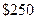The marginal cost of producing computers isfor the first computer,for the second,for the third,for the fourth,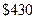for the fifth,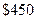for the sixth, and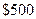for the seventh.a. Create a table that shows the company’s output, total cost, marginal cost, average cost, variable cost, and average variable cost.b. At what price is the zero-profit point? At what price is the shutdown point?c. If the company sells the computers foris it making a profit or a loss? How big is the profit or loss? Sketch a graph with AC, MC, and AVG curves to illustrate your answer and show the profit or loss.d. If the firm sells the computers for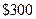is it making a profit or a loss? How big is the profit or loss? Sketch a graph with AC, MC, and AVG curves to illustrate your answer and show the profit or loss.

a.

To determine

A table showing total output, total cost, marginal cost, average cost, variable cost, and average variable cost.

Explanation

Using the given data, we have calculated the required figures corresponding to total output, total cost, marginal cos...

### Still sussing out bartleby?

Check out a sample textbook solution.

See a sample solution

#### The Solution to Your Study Problems

Bartleby provides explanations to thousands of textbook problems written by our experts, many with advanced degrees!

Get Started